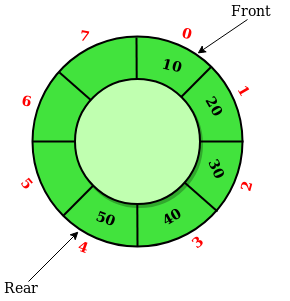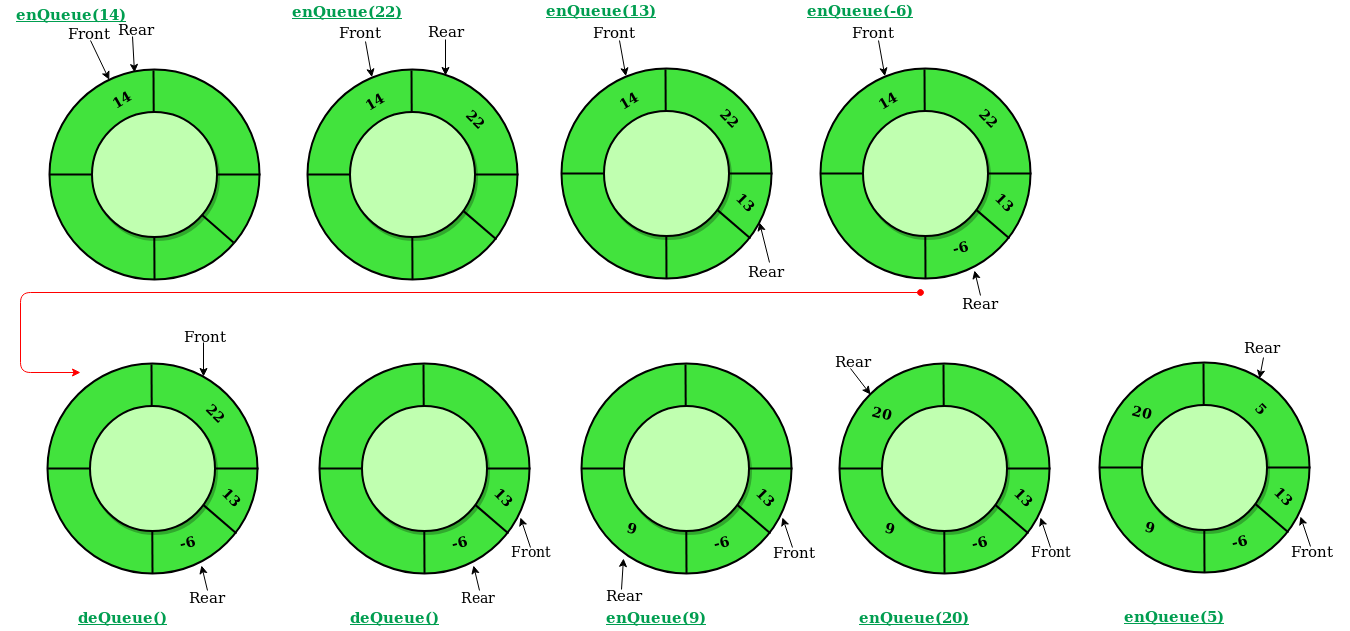Circular Queue | Set 1 (Introduction and Array Implementation)

• Difficulty Level : Easy
• Last Updated : 31 Aug, 2021

Prerequisite – Queues
Circular Queue is a linear data structure in which the operations are performed based on FIFO (First In First Out) principle and the last position is connected back to the first position to make a circle. It is also called ‘Ring Buffer’In a normal Queue, we can insert elements until queue becomes full. But once queue becomes full, we can not insert the next element even if there is a space in front of queue.Operations on Circular Queue:

• Front: Get the front item from queue.
• Rear: Get the last item from queue.
• enQueue(value) This function is used to insert an element into the circular queue. In a circular queue, the new element is always inserted at Rear position.
1. Check whether queue is Full – Check ((rear == SIZE-1 && front == 0) || (rear == front-1)).
2. If it is full then display Queue is full. If queue is not full then, check if (rear == SIZE – 1 && front != 0) if it is true then set rear=0 and insert element.
• deQueue() This function is used to delete an element from the circular queue. In a circular queue, the element is always deleted from front position.
1. Check whether queue is Empty means check (front==-1).
2. If it is empty then display Queue is empty. If queue is not empty then step 3
3. Check if (front==rear) if it is true then set front=rear= -1 else check if (front==size-1), if it is true then set front=0 and return the element.

C++

 // C or C++ program for insertion and// deletion in Circular Queue#includeusing namespace std; class Queue{    // Initialize front and rear    int rear, front;     // Circular Queue    int size;    int *arr;     Queue(int s)    {       front = rear = -1;       size = s;       arr = new int[s];    }     void enQueue(int value);    int deQueue();    void displayQueue();};  /* Function to create Circular queue */void Queue::enQueue(int value){    if ((front == 0 && rear == size-1) ||            (rear == (front-1)%(size-1)))    {        printf("\nQueue is Full");        return;    }     else if (front == -1) /* Insert First Element */    {        front = rear = 0;        arr[rear] = value;    }     else if (rear == size-1 && front != 0)    {        rear = 0;        arr[rear] = value;    }     else    {        rear++;        arr[rear] = value;    }} // Function to delete element from Circular Queueint Queue::deQueue(){    if (front == -1)    {        printf("\nQueue is Empty");        return INT_MIN;    }     int data = arr[front];    arr[front] = -1;    if (front == rear)    {        front = -1;        rear = -1;    }    else if (front == size-1)        front = 0;    else        front++;     return data;} // Function displaying the elements// of Circular Queuevoid Queue::displayQueue(){    if (front == -1)    {        printf("\nQueue is Empty");        return;    }    printf("\nElements in Circular Queue are: ");    if (rear >= front)    {        for (int i = front; i <= rear; i++)            printf("%d ",arr[i]);    }    else    {        for (int i = front; i < size; i++)            printf("%d ", arr[i]);         for (int i = 0; i <= rear; i++)            printf("%d ", arr[i]);    }} /* Driver of the program */int main(){    Queue q(5);     // Inserting elements in Circular Queue    q.enQueue(14);    q.enQueue(22);    q.enQueue(13);    q.enQueue(-6);     // Display elements present in Circular Queue    q.displayQueue();     // Deleting elements from Circular Queue    printf("\nDeleted value = %d", q.deQueue());    printf("\nDeleted value = %d", q.deQueue());     q.displayQueue();     q.enQueue(9);    q.enQueue(20);    q.enQueue(5);     q.displayQueue();     q.enQueue(20);    return 0;}

Java

 // Java program for insertion and// deletion in Circular Queueimport java.util.ArrayList; class CircularQueue{ // Declaring the class variables.private int size, front, rear; // Declaring array list of integer type.private ArrayList queue = new ArrayList(); // ConstructorCircularQueue(int size){    this.size = size;    this.front = this.rear = -1;} // Method to insert a new element in the queue.public void enQueue(int data){         // Condition if queue is full.    if((front == 0 && rear == size - 1) ||      (rear == (front - 1) % (size - 1)))    {        System.out.print("Queue is Full");    }     // condition for empty queue.    else if(front == -1)    {        front = 0;        rear = 0;        queue.add(rear, data);    }     else if(rear == size - 1 && front != 0)    {        rear = 0;        queue.set(rear, data);    }     else    {        rear = (rear + 1);             // Adding a new element if        if(front <= rear)        {            queue.add(rear, data);        }             // Else updating old value        else        {            queue.set(rear, data);        }    }} // Function to dequeue an element// form th queue.public int deQueue(){    int temp;     // Condition for empty queue.    if(front == -1)    {        System.out.print("Queue is Empty");                 // Return -1 in case of empty queue        return -1;    }     temp = queue.get(front);     // Condition for only one element    if(front == rear)    {        front = -1;        rear = -1;    }     else if(front == size - 1)    {        front = 0;    }    else    {        front = front + 1;    }         // Returns the dequeued element    return temp;} // Method to display the elements of queuepublic void displayQueue(){         // Condition for empty queue.    if(front == -1)    {        System.out.print("Queue is Empty");        return;    }     // If rear has not crossed the max size    // or queue rear is still greater then    // front.    System.out.print("Elements in the " +                     "circular queue are: ");     if(rear >= front)    {             // Loop to print elements from        // front to rear.        for(int i = front; i <= rear; i++)        {            System.out.print(queue.get(i));            System.out.print(" ");        }        System.out.println();    }     // If rear crossed the max index and    // indexing has started in loop    else    {                 // Loop for printing elements from        // front to max size or last index        for(int i = front; i < size; i++)        {            System.out.print(queue.get(i));            System.out.print(" ");        }         // Loop for printing elements from        // 0th index till rear position        for(int i = 0; i <= rear; i++)        {            System.out.print(queue.get(i));            System.out.print(" ");        }        System.out.println();    }} // Driver codepublic static void main(String[] args){         // Initialising new object of    // CircularQueue class.    CircularQueue q = new CircularQueue(5);         q.enQueue(14);    q.enQueue(22);    q.enQueue(13);    q.enQueue(-6);         q.displayQueue();     int x = q.deQueue();     // Checking for empty queue.    if(x != -1)    {        System.out.print("Deleted value = ");        System.out.println(x);    }     x = q.deQueue();     // Checking for empty queue.    if(x != -1)    {        System.out.print("Deleted value = ");        System.out.println(x);    }     q.displayQueue();         q.enQueue(9);    q.enQueue(20);    q.enQueue(5);         q.displayQueue();         q.enQueue(20);}} // This code is contributed by Amit Mangal.

Python 3

 class CircularQueue():     # constructor    def __init__(self, size): # initializing the class        self.size = size                 # initializing queue with none        self.queue = [None for i in range(size)]        self.front = self.rear = -1     def enqueue(self, data):                 # condition if queue is full        if ((self.rear + 1) % self.size == self.front):            print(" Queue is Full\n")                     # condition for empty queue        elif (self.front == -1):            self.front = 0            self.rear = 0            self.queue[self.rear] = data        else:                         # next position of rear            self.rear = (self.rear + 1) % self.size            self.queue[self.rear] = data                 def dequeue(self):        if (self.front == -1): # condition for empty queue            print ("Queue is Empty\n")                     # condition for only one element        elif (self.front == self.rear):            temp=self.queue[self.front]            self.front = -1            self.rear = -1            return temp        else:            temp = self.queue[self.front]            self.front = (self.front + 1) % self.size            return temp     def display(self):             # condition for empty queue        if(self.front == -1):            print ("Queue is Empty")         elif (self.rear >= self.front):            print("Elements in the circular queue are:",                                              end = " ")            for i in range(self.front, self.rear + 1):                print(self.queue[i], end = " ")            print ()         else:            print ("Elements in Circular Queue are:",                                           end = " ")            for i in range(self.front, self.size):                print(self.queue[i], end = " ")            for i in range(0, self.rear + 1):                print(self.queue[i], end = " ")            print ()         if ((self.rear + 1) % self.size == self.front):            print("Queue is Full") # Driver Codeob = CircularQueue(5)ob.enqueue(14)ob.enqueue(22)ob.enqueue(13)ob.enqueue(-6)ob.display()print ("Deleted value = ", ob.dequeue())print ("Deleted value = ", ob.dequeue())ob.display()ob.enqueue(9)ob.enqueue(20)ob.enqueue(5)ob.display() # This code is contributed by AshwinGoel

Output:

Elements in Circular Queue are: 14 22 13 -6
Deleted value = 14
Deleted value = 22
Elements in Circular Queue are: 13 -6
Elements in Circular Queue are: 13 -6 9 20 5
Queue is Full

Time Complexity: Time complexity of enQueue(), deQueue() operation is O(1) as there is no loop in any of the operation.
Applications:

1. Memory Management: The unused memory locations in the case of ordinary queues can be utilized in circular queues.
2. Traffic system: In computer controlled traffic system, circular queues are used to switch on the traffic lights one by one repeatedly as per the time set.
3. CPU Scheduling: Operating systems often maintain a queue of processes that are ready to execute or that are waiting for a particular event to occur.